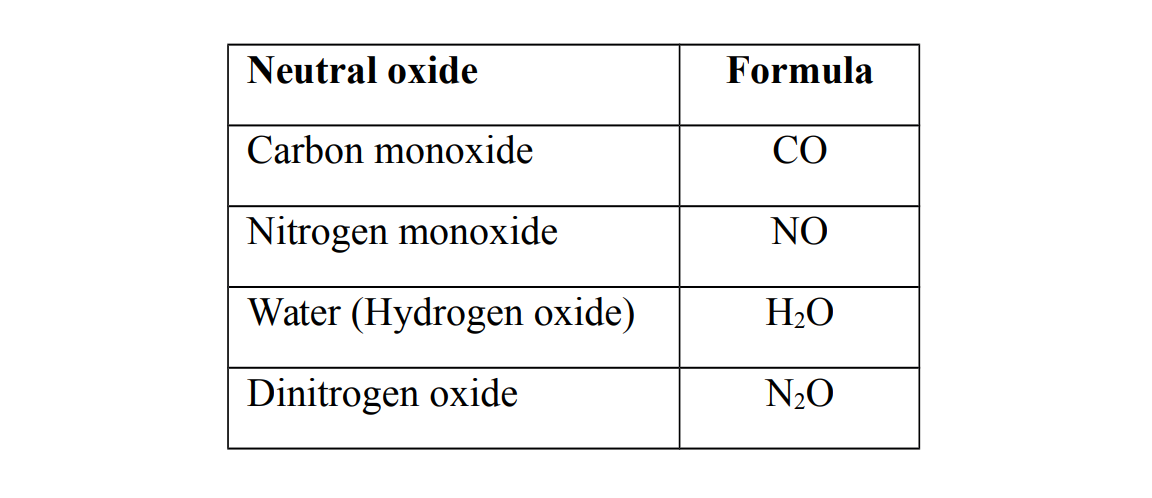# Acids

An acid is a chemical substance which when dissolved in water produces hydrogen. An acid can also be defined as a proton donor.

Note The hydrogen ions give an acid its characteristic properties.

#### Types of acids

Acids can be classified into two categories

##### 1. Mineral acids

Mineral acids are also called inorganic acids

They are acids that are prepared from the minerals obtained from the earth

Generally all mineral acids are strong acids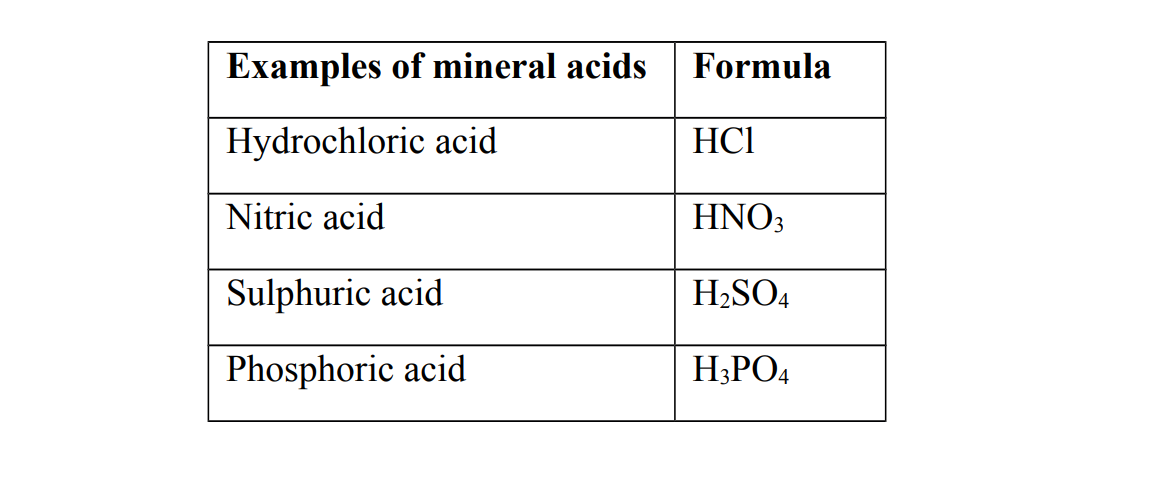##### Organic acids

Organic acids are naturally occurring acids

They are found in organic matter of living things. They are prepared from organic sources such as plants

Generally organic acids are weak acids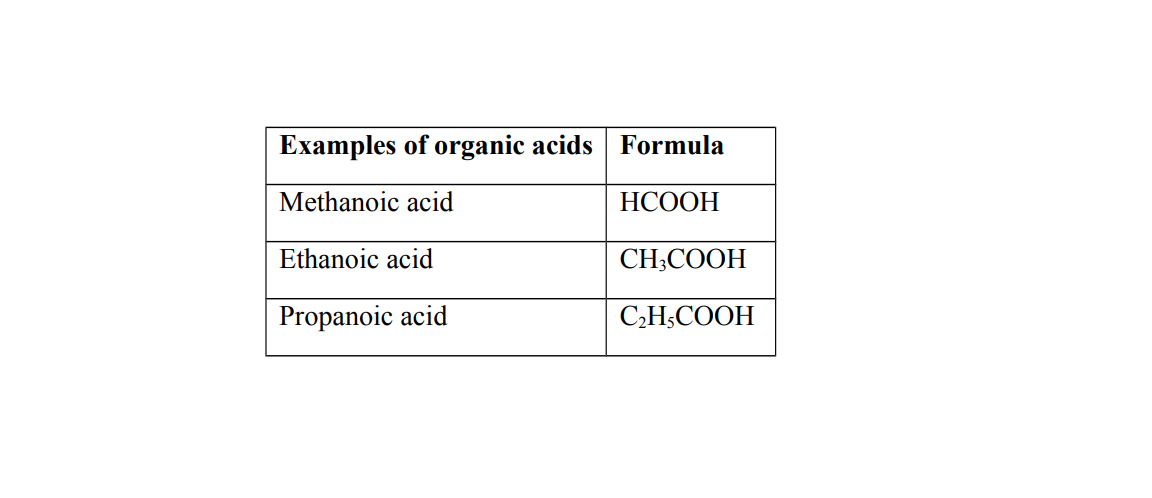##### Ionization of acids

Ionization is the process of forming ions

An ion is a charged particle

When acids dissolve in water, they produce hydrogen ions, H+ , as the only positively charged ions

##### Examples of ionization of acids

(a) HCl(aq) → ${H}^{+}$ (aq) + ${\mathrm{Cl}}^{-}$ (aq)

(b) ${\mathrm{HNO}}_{3}$ (aq) → ${H}^{+}$ (aq) + ${\mathrm{{\mathrm{NO}}_{3}}}^{-}$ (aq)

#### Strength of acids

Strength of an acid is the measure of its ability to produce hydrogen ions. It can also be defined as the ability to donate protons. Acids can be grouped into two classes

##### Weak acids

A weak acid is an acid that partially ionizes when dissolved in water

Weak acids have reversible ionization

##### Examples of weak acids

(a) Ethanoic acid (b) Carbonic acid

##### Strong acids

A strong is an acid that completely ionizes when dissolved in water

##### Examples of strong acids

(a) Hydrochloric acid (b) Nitric acid (c) Sulphuric acid (d) Phosphoric acid

#### Basicity of an acid

Basicity of an acid is the number of moles of hydrogen ions, H+ , produced from one mole of an acid. Alternative term: Protocity of an acid.

##### 1. Monobasic acid

A monobasic acid is an acid that produces one hydrogen ion, e.g Hydrochloric acid, Nitric acid and Ethanoic acid

##### 2. Dibasic acid

A dibasic acid is an acid that produces two hydrogen ions, e.g Carbonic acid, Sulphuric acid

##### 3. Tribasic acid

A tribasic is an acid is an acid that produces three hydrogen ions, e.g Phosphoric acid

##### Physical properties of acids
1. Acids have PH numbers less than 7
2. Acids turn blue litmus paper red
3. Acids have a sour taste
##### Chemical properties of acids

1. Acids react with reactive metals to form a salt and hydrogen gas, H2

Note Metals below hydrogen in the reactivity series cannot react with acids

2. Acids react with bases and alkalis to form a salt and water only. The reaction between an acid and a base is called neutralization .

Acids react with carbonates or hydrogen carbonates to form a salt, water and carbon dioxide gas

#### Bases

A base is a substance which reacts with an acid to form a salt and water. A base can also be defined as an oxide or hydroxide of a metal or a proton acceptor

A base neutralizes an acid. A soluble base is called an alkali

An alkali is a substance which when dissolved in water produces the hydroxide ions, OH- , as the only negatively charged ions. Examples of alkalis are Sodium hydroxide, Potassium hydroxide, Ammonium hydroxide and Calcium hydroxide

Examples of insoluble bases are Copper (II) oxide, Zinc oxide, Iron (III) oxide and Lead (II) hydroxide

#### Ionization of alkalis

When alkalis dissolve in water, they produce hydroxide ions, OH- , as the only negatively charged ions.

#### Examples of ionization of alkalis

(a) NaOH(aq) → ${\mathrm{Na}}^{+}$ (aq) + ${\mathrm{OH}}^{-}$ (aq)

(a) KOH(aq) → ${k}^{+}$ (aq) + ${\mathrm{OH}}^{-}$ (aq)

##### Strength of alkalis

Strength of an alkali is the measure of its ability to produce hydroxide ions

Alkalis can be classified into two categories

##### 1. Weak alkalis

A weak alkali is an alkali that partially ionizes when dissolved in water

Weak alkalis have reversible ionization

##### Example of a weak alkali

(a) Ammonium hydroxide

##### 2. Strong alkalis

A strong alkali is an alkali that ionizes completely when dissolved in water

Examples of strong acids are (a) Sodium hydroxide (b) Potassium hydroxide (c) Calcium hydroxide

##### Physical properties of alkalis
1. Alkalis have PH numbers greater than 7
2. Alkalis turn red litmus paper blue
3. Alkalis have a bitter taste and feel soapy or slippery between fingers
##### Chemical properties of bases and alkalis
1. Alkalis and bases react with acids to form a salt and water only
2. Alkalis reacts with ammonium compounds to form a salt, water and ammonia gas
3. Alkalis react with solutions of soluble salts to form precipitates of insoluble hydroxides
4. Alkalis react with acidic oxides to form a salt and water only

#### The PH scale

PH is the degree of acidity or alkalinity of a substance

PH stands for Potenz Hydrogen. Potenz is a German term which means potential

The PH scale ranges from 0 to 14

PH values from 0 to 7 implies acidic medium.

PH values 7 imply a neutral medium e.g. water, H2O and sodium chloride, NaCl

PH values from 7 to 14 implies alkaline medium

The lower the PH value, the stronger the acidity e.g. hydrochloric acid (HCl), sulphuric acid (H2SO4) and nitric acid (HNO3)

The greater the PH value, the stronger the alkalinity e.g. Potassium hydroxide (KOH) and sodium hydroxide (NaOH)

The ion responsible for acidity is the hydrogen ion, H+ and the ion responsible for alkalinity is the hydroxide ion, OH-

PH is a numerical value and has no units

PH is related to the concentration of acids and alkalis.

#### Indicators

An indicator is a chemical substance which has different colours with different substances or solutions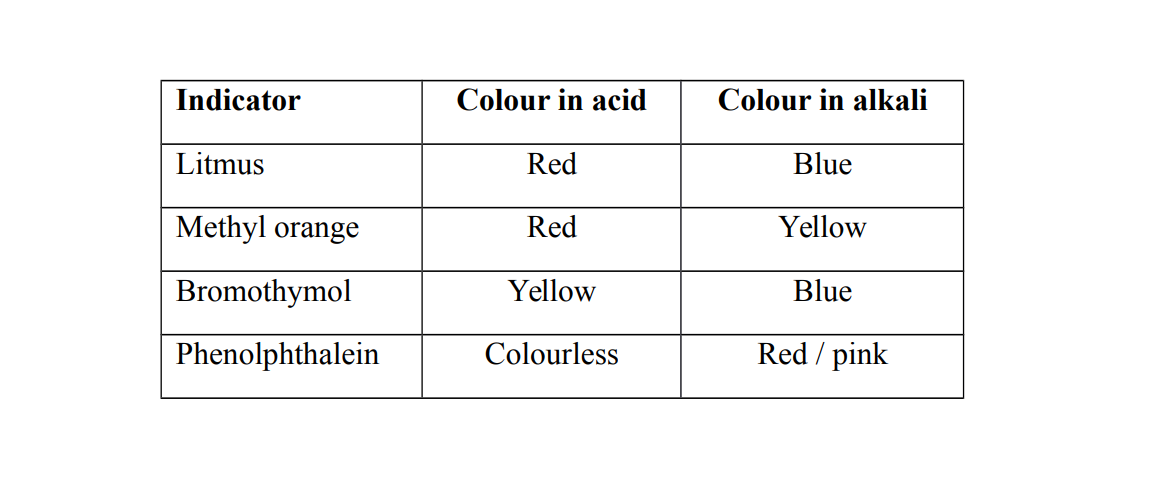#### Universal indicator

A universal indicator is a solution or paper which shows different colors in acidic or alkaline conditions

$\begin{array}{|llll|}\hline PH scale& 1,2,3& 4,5& 6\\ Color& Red& Orange& Yellow\\ \hline\end{array}$ $\begin{array}{|lllll|}\hline PH scale& 7& 8& 9, 10& 11, 12, 13, 14\\ Color& Green& Blue& Indigo& Purple\\ \hline\end{array}$
##### Soil acidity
###### Formation of acid rain

Acid rain is formed when gaseous acidic oxides dissolve in rain water in the atmosphere

##### What is destroyed by acid rain?

1. Soil – the soil becomes acidic

2. Crops and vegetation

##### Examples of acidic rain fall

1. Nitric acid is formed when nitrogen dioxide gas dissolve in rain water in the atmosphere

NO2(g) + H2O(l) → HNO3(aq)

2. Carbonic acid is formed when carbon dioxide gas dissolve in rain water in the atmosphere

CO2(g) + H2O(l) → H2CO3(aq)

3 . Sulphuric acid is formed when sulphur dioxide gas dissolve in rain water in the atmosphere

SO2(g) + H2O(l) → H2SO4(aq)

All these acids come down as acid rainfall. Acid rain causes pollution and Acidic soils on the farm can be neutralized or avoided by adding lime (Calcium oxide)

#### Oxide

An oxide is a compound formed when oxygen combines with any other element

#### Classification of oxides

Acidic oxides are oxides of non-metals. They are formed when a non – metal reacts with oxygen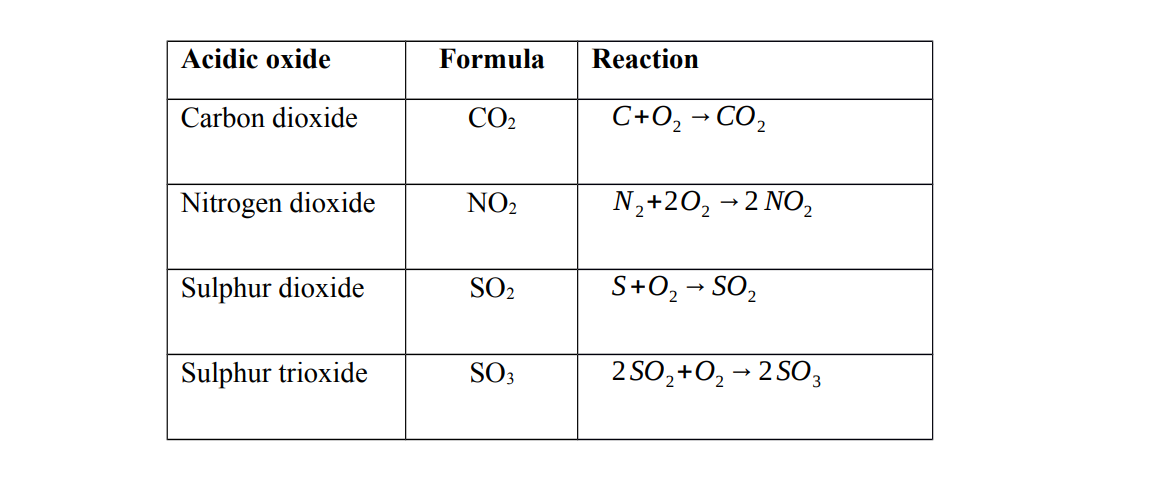##### Characteristics of acidic oxides

1. Acidic oxides dissolve in water to form acids

Note Dinitrogen tetra oxide forms two acids when dissolved in water

They are called acidic oxides because they react with water to form acids

2. Acidic oxides react with alkalis to form a salt and water only

##### Basic oxides

Basic oxides are oxides of metals. They are formed when a metal reacts with oxygen

##### Characteristics of basic oxides

1. Some soluble basic oxides dissolve in water to form alkalis

2. Basic oxides react with acids to form a salt and water only

##### Amphoteric oxides

Amphoteric oxides are oxides of metals. Amphoteric oxides show both basic and acidic properties i.e. they react with both acids and alkalis to form a salt and water

(a) Reaction with acids (b) Reaction with alkalis

##### Neutral oxides

Neutral oxides do not show either basic or acidic properties i.e. they do not react with either bases or acids